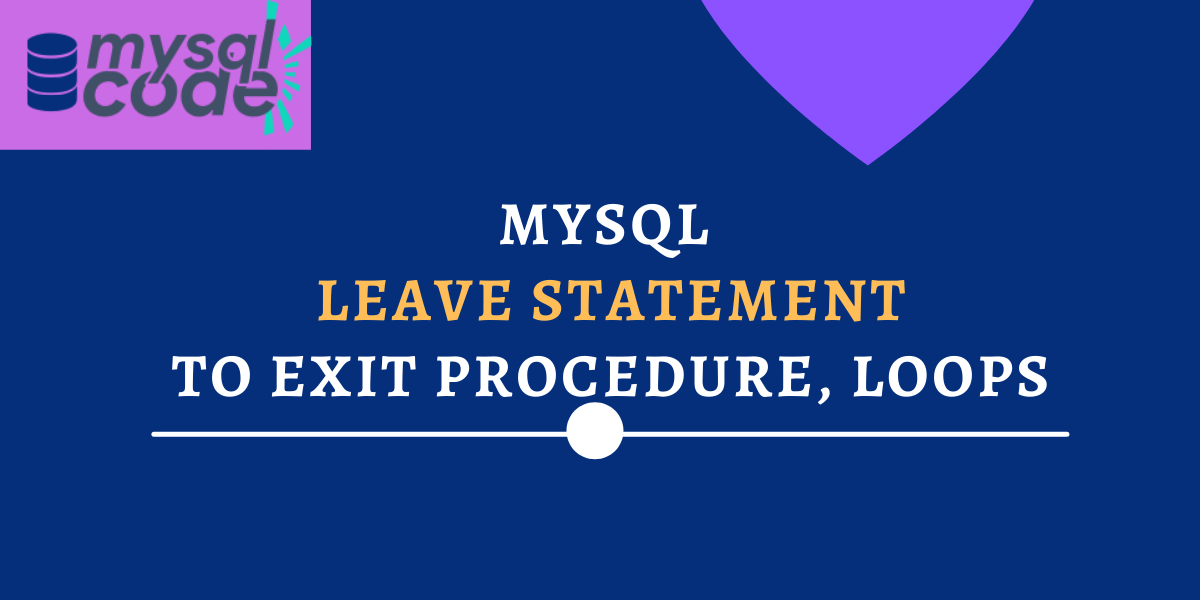# MySQL LEAVE Statement – Terminate Stored Procedures, Functions and LoopsDid you ever try to exit the loop or block of code in MySQL and struggle to achieve so? If yes then this tutorial will help you in an easy way. In this tutorial, we will learn how to exit from the block of code or break the block of code in MySQL with the help of the LEAVE statement. This is a very helpful element in MySQL that very few people know about. So, we will study it with syntax and an example.

## Introduction to MySQL LEAVE Statement

By definition, the MySQL LEAVE statement is used to break the flow control of the block of code that has the given label. It is mainly used for stored programs and if the label is specified for the outermost block, it exits the program.

The LEAVE statement is used within the BEGIN…END block or loops such as WHILE, LOOP and REPEAT. It is exactly the same as the break statement available in many programming languages such as Java, C and C++.

## Syntax to use MySQL LEAVE Statement

The LEAVE statement can be used inside the loops or begin-end blocks with the help of labels. The following block of code shows how can we use the LEAVE statement in MySQL.

### Syntax 1- LEAVE Statement with BEGIN-END Block

```.wp-block-code {
border: 0;
}

.wp-block-code > span {
display: block;
overflow: auto;
}

.shcb-language {
border: 0;
clip: rect(1px, 1px, 1px, 1px);
-webkit-clip-path: inset(50%);
clip-path: inset(50%);
height: 1px;
margin: -1px;
overflow: hidden;
position: absolute;
width: 1px;
word-wrap: normal;
word-break: normal;
}

.hljs {
box-sizing: border-box;
}

.hljs.shcb-code-table {
display: table;
width: 100%;
}

.hljs.shcb-code-table > .shcb-loc {
color: inherit;
display: table-row;
width: 100%;
}

.hljs.shcb-code-table .shcb-loc > span {
display: table-cell;
}

.wp-block-code code.hljs:not(.shcb-wrap-lines) {
white-space: pre;
}

.wp-block-code code.hljs.shcb-wrap-lines {
white-space: pre-wrap;
}

.hljs.shcb-line-numbers {
border-spacing: 0;
counter-reset: line;
}

.hljs.shcb-line-numbers > .shcb-loc {
counter-increment: line;
}

.hljs.shcb-line-numbers .shcb-loc > span {
}

.hljs.shcb-line-numbers .shcb-loc::before {
border-right: 1px solid #ddd;
content: counter(line);
display: table-cell;
text-align: right;
-webkit-user-select: none;
-moz-user-select: none;
-ms-user-select: none;
user-select: none;
white-space: nowrap;
width: 1%;
}```CREATE PROCEDURE proc()
block_label: BEGIN
IF condition THEN
LEAVE block_label;
END IF;
other_statements
END\$\$
```Code language: SQL (Structured Query Language) (sql)```

Where,

• proc() – It is a procedure created using the CREATE PROCEDURE statement.
• block_label – It is a name that is given to the block. You can give labels to loops as well as begin-end blocks.
• LEAVE block_label – It terminates the block when a certain condition becomes true and passes the flow control outside of the block

### Syntax 2- LEAVE Statement with Loops

As mentioned earlier, we can use the LEAVE statement with MySQL loops as well such as LOOP, REPEAT and WHILE.

``````[label]: LOOP/REPEAT/WHILE
IF condition THEN
LEAVE [label];
END IF;
-- statements
[UNTIL search_condition (only for REPEAT loop)]
END LOOP/REPEAT/WHILE [label];```Code language: SQL (Structured Query Language) (sql)```

“UNTIL search_condition” is used only for the REPEAT loop.

In the above syntax, you can see that the label is optional. Whereas, when you write the BEGIN-END block, you must specify the label.

## Examples of MySQL LEAVE Statement

Now we will see an example to understand the LEAVE statement effectively.

We will create a stored procedure of the name “leaveExample” and write a LOOP loop. We will accept two values from the user as parameters to the procedure.

If the first value is smaller than the second value then we will increment the first value till the second value and print all of them. On the other hand, If the first value is larger than the second value then we will decrement the first value till the second value and print them.

``````DELIMITER //
CREATE PROCEDURE leaveExample(IN x INT,IN y INT)
BEGIN
DECLARE outp VARCHAR(100) DEFAULT ' ';

IF(x>y) THEN
decr:LOOP
SET outp = CONCAT(outp,x,',');
IF(x=y) THEN
LEAVE decr;
END IF;
SET x= x-1;
END LOOP;

ELSEIF(x<y) THEN
incr:LOOP
SET outp = CONCAT(outp,x,',');
IF(x=y) THEN
LEAVE incr;
END IF;
SET x=x+1;
END LOOP;

Else
SET outp="Both are Equal, can't iterate";

END IF;
SELECT outp as output;
END //```Code language: SQL (Structured Query Language) (sql)```

Explanation-

• In the above example, we have used the IF-THEN-ELSE statement to check whether the first number is greater or second.
• If the first number is greater, we will start the loop of label “decr”. We use the CONCAT() function to concatenate all numbers into a single string.
• Then we again use the IF condition to check if both numbers become similar.
• We then decrement the value of x until it becomes the same as y. If the x becomes the same as y, we will break the loop using the LEAVE statement. This way, the control goes out of the loop and then the outer IF-THEN-ELSE block ends.
• Similarly, if the first number is smaller, we will start the loop of label “incr” to increment the value of x and perform the similar operation as mentioned above.

Now let’s test the procedure to check if it works correctly.

``CALL leaveExample(1,10);`Code language: SQL (Structured Query Language) (sql)`

Here you can see, we have received the output of numbers 1 to 10.

Let’s pass the same parameters in reverse order.

``CALL leaveExample(10,1);`Code language: SQL (Structured Query Language) (sql)`

As you can see, we got numbers 1 to 10 in reverse order.

Now let’s pass the two same parameters and see what we get.

``CALL leaveExample(10,10);`Code language: SQL (Structured Query Language) (sql)`